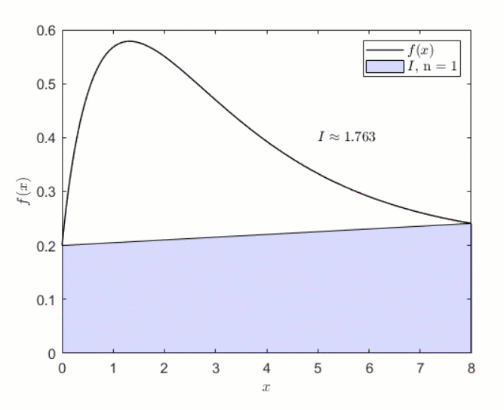## Numerical Integration

버전 1.0.1 (67.7 KB) 작성자:
Interactive courseware module that addresses the fundamentals of numerical integration taught in mathematics courses.

다운로드 수: 164

업데이트 날짜: 2021/6/28

GitHub에서 호스트

# Numerical IntegrationCurriculum Module created in collaboration with Dr. Matthew Leineweber, San Jose State University.

Created with R2020b. Compatible with R2020b and later releases.## Description

This curriculum module contains interactive live scripts that teach two fundamental techniques for approximating definite integrals: the trapezoid and Simpson’s rules. These rules are derived from Lagrange interpolating polynomials and explored through interactive visualizations. Each lesson concludes with a guided activity in which students implement the discussed integration rule. These live scripts can be used as part of a lecture, as activities in an instructional setting, or as an interactive assignment to be completed outside of class.

The instructions inside the live scripts will guide you through the exercises and activities. Get started with each live script by running it one section at a time. To stop running the script or a section midway (for example, when an animation is in progress), use the Stop button in the RUN section of the Live Editor tab in the MATLAB Toolstrip.

## Suggested Prework

MATLAB Onramp – a free two-hour introductory tutorial that teaches the essentials of MATLAB.

## Details

`TrapezoidRule.mlx, TrapezoidRuleSoln.mlx`
An interactive lesson that explores the trapezoid rule.

Learning Goals:

• Explain numerical quadrature and its relationship to the definite integral
• Describe how the trapezoid rule is derived
• Illustrate the trapezoid rule graphically
• Compare and contrast integration of a continuous function with integration of tabulated data
• Implement the trapezoid rule in MATLAB

`SimpsonsRules.mlx, SimpsonsRulesSoln.mlx`
An interactive lesson that explores Simpson’s rules.

Learning Goals:

• Describe how Lagrange interpolating polynomials can be used to derive integration rules
• Illustrate Simpson’s 1/3 rule graphically
• Explain the steps required to implement Simpson’s 1/3 rule
• Compare the accuracy and limitations of Simpson’s 1/3 rule to those of Simpson’s 3/8 rule
• Implement Simpson’s 3/8 rule in MATLAB

## Products

MATLAB®

The license for this module is available in the LICENSE.TXT file in this GitHub repository.

## Educator Resources

Have any questions or feedback? Contact the MathWorks online teaching team.

### 인용 양식

Brian Hong (2022). Numerical Integration (https://github.com/MathWorks-Teaching-Resources/Numerical-Integration/releases/tag/v1.0.1), GitHub. 검색됨 .

##### MATLAB 릴리스 호환 정보
개발 환경: R2020b
R2020b 이상 릴리스와 호환
##### 플랫폼 호환성
Windows macOS Linux
##### 커뮤니티
Distance Learning 커뮤니티의 더 많은 파일

### Community Treasure Hunt

Find the treasures in MATLAB Central and discover how the community can help you!

Start Hunting!
이 GitHub 애드온의 문제를 보거나 보고하려면 GitHub 리포지토리로 가십시오.
이 GitHub 애드온의 문제를 보거나 보고하려면 GitHub 리포지토리로 가십시오.